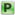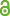Hauptmenü
• Autor
• Berkes, István
• Tichy, Robert
• TitelLacunary series and stable distributions
• Datei
• DOI10.1007/978-3-319-12442-1_2
• Erschienen inMathematical Statistics and Limit Theorems
• Erscheinungsjahr2015
• Seiten7-19
• LicenceCC BY
• ISBN978-3-319-12442-1
• Zugriffsrechte• AbstractBy well-known results of probability theory, any sequence of random variables with bounded second moments has a subsequence satisfying the central limit theorem and the law of the iterated logarithm in a randomized form. In this paper we give criteria for a sequence $$(X_n)$$ of random variables to have a subsequence $$(X_{n_k})$$ whose weighted partial sums, suitably normalized, converge weakly to a symmetric stable distribution with parameter $$0<\alpha <2$$.